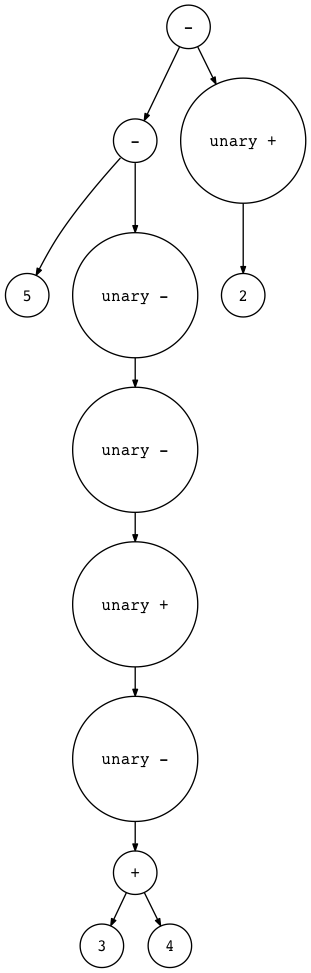# 一起来写个简单的解释器（8）

• 扩展语法来处理一元加号和一元减号运算符
• 添加一个新的 UnaryOp AST节点类
• 扩展解析器以生成带有UnaryOp节点的AST
• 扩展解释器并添加一个新的visit_UnaryOp方法来解释一元运算符

• 一元减号 (-) 运算符对其数字操作数的求反
• 一元加 (+) 运算符产生的数字操作数没有改变
• 一元运算符的优先级高于二元运算符 +， - ，*和/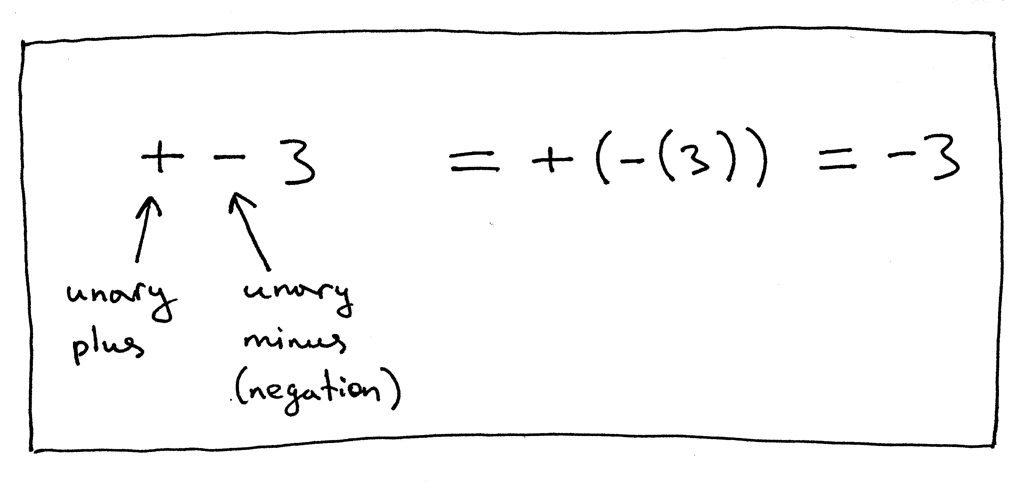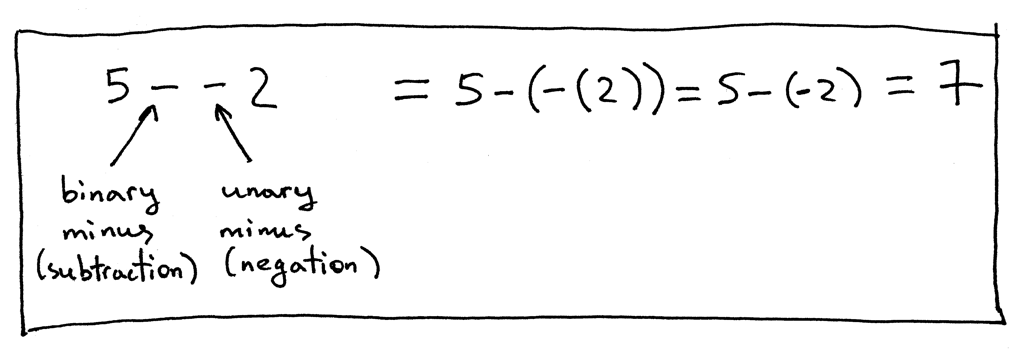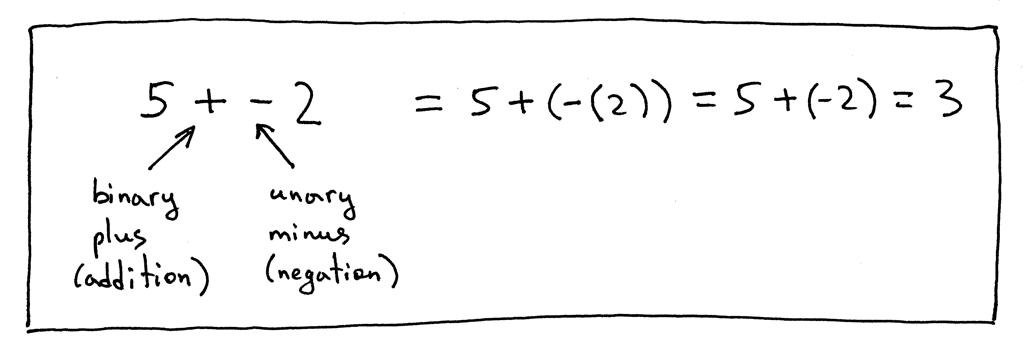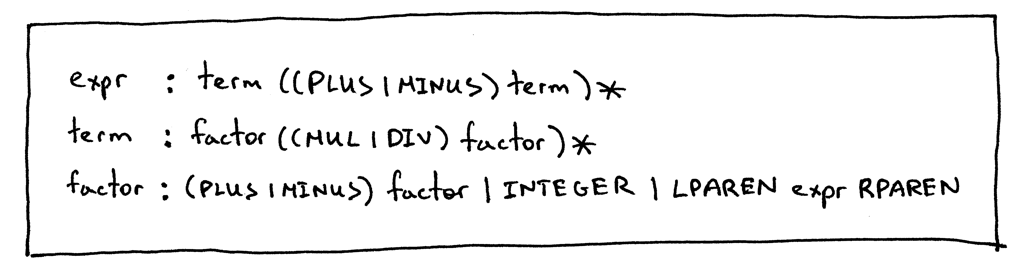``````class UnaryOp(AST):
def __init__(self, op, expr):
self.token = self.op = op
self.expr = expr
``````

``````def factor(self):
"""factor : (PLUS | MINUS) factor | INTEGER | LPAREN expr RPAREN"""
token = self.current_token
if token.type == PLUS:
self.eat(PLUS)
node = UnaryOp(token, self.factor())
return node
elif token.type == MINUS:
self.eat(MINUS)
node = UnaryOp(token, self.factor())
return node
elif token.type == INTEGER:
self.eat(INTEGER)
return Num(token)
elif token.type == LPAREN:
self.eat(LPAREN)
node = self.expr()
self.eat(RPAREN)
return node
``````

``````def visit_UnaryOp(self, node):
op = node.op.type
if op == PLUS:
return +self.visit(node.expr)
elif op == MINUS:
return -self.visit(node.expr)
``````

``````>>> from spi import BinOp, UnaryOp, Num, MINUS, INTEGER, Token
>>> five_tok = Token(INTEGER, 5)
>>> two_tok = Token(INTEGER, 2)
>>> minus_tok = Token(MINUS, '-')
>>> expr_node = BinOp(
...     Num(five_tok),
...     minus_tok,
...     UnaryOp(minus_token, UnaryOp(minus_token, Num(two_tok)))
... )
>>> from spi import Interpreter
>>> inter = Interpreter(None)
>>> inter.visit(expr_node)
3
``````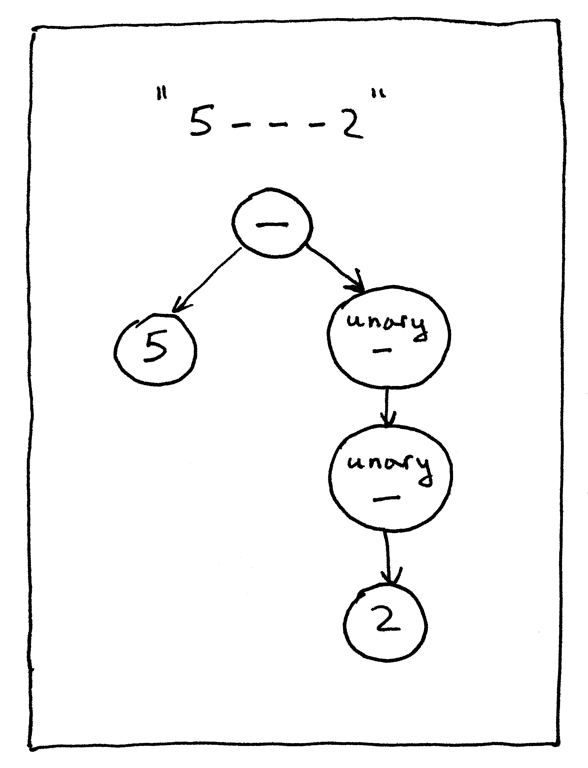``````\$ python spi.py
spi> - 3
-3
spi> + 3
3
spi> 5 - - - + - 3
8
spi> 5 - - - + - (3 + 4) - +2
10
``````

``````\$ python genastdot.py "- 3" > ast.dot && dot -Tpng -o ast.png ast.dot
``````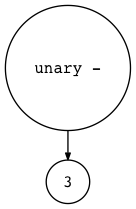``````\$ python genastdot.py "+ 3" > ast.dot && dot -Tpng -o ast.png ast.dot
``````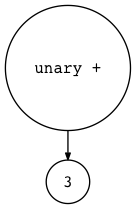``````\$ python genastdot.py "5 - - - + - 3" > ast.dot && dot -Tpng -o ast.png ast.dot
``````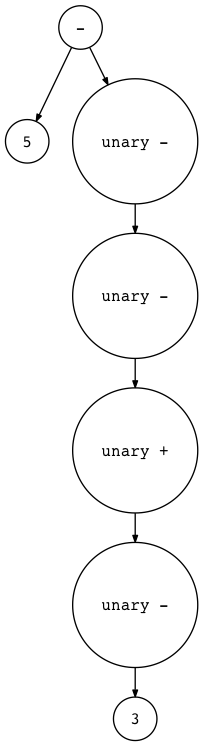``````\$ python genastdot.py "5 - - - + - (3 + 4) - +2" \
> ast.dot && dot -Tpng -o ast.png ast.dot
``````